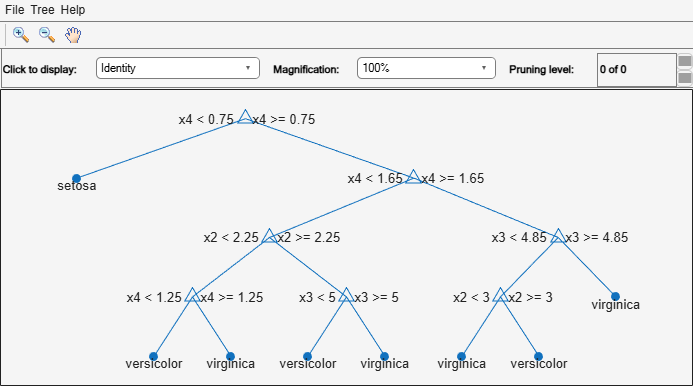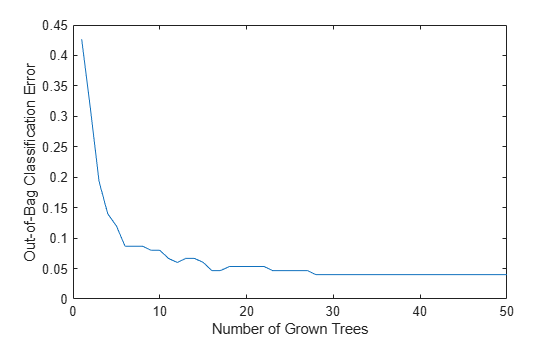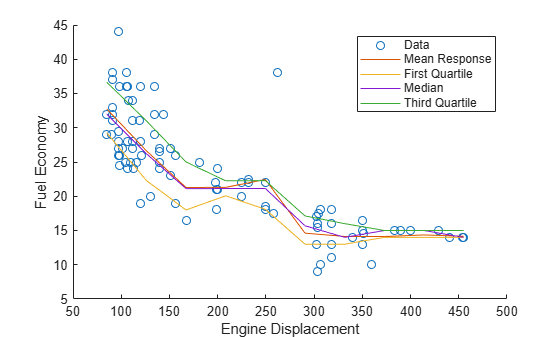# TreeBagger class

Bag of decision trees

## Description

`TreeBagger` bags an ensemble of decision trees for either classification or regression. Bagging stands for bootstrap aggregation. Every tree in the ensemble is grown on an independently drawn bootstrap replica of input data. Observations not included in this replica are "out of bag" for this tree.

`TreeBagger` relies on the `ClassificationTree` and `RegressionTree` functionality for growing individual trees. In particular, `ClassificationTree` and `RegressionTree` accepts the number of features selected at random for each decision split as an optional input argument. That is, `TreeBagger` implements the random forest algorithm .

For regression problems, `TreeBagger` supports mean and quantile regression (that is, quantile regression forest ).

## Construction

 TreeBagger Create bag of decision trees

## Object Functions

 `append` Append new trees to ensemble `compact` Compact ensemble of decision trees `error` Error (misclassification probability or MSE) `fillprox` Proximity matrix for training data `growTrees` Train additional trees and add to ensemble `margin` Classification margin `mdsprox` Multidimensional scaling of proximity matrix `meanMargin` Mean classification margin `oobError` Out-of-bag error `oobMargin` Out-of-bag margins `oobMeanMargin` Out-of-bag mean margins `oobPredict` Ensemble predictions for out-of-bag observations `oobQuantileError` Out-of-bag quantile loss of bag of regression trees `oobQuantilePredict` Quantile predictions for out-of-bag observations from bag of regression trees `partialDependence` Compute partial dependence `plotPartialDependence` Create partial dependence plot (PDP) and individual conditional expectation (ICE) plots `predict` Predict responses using ensemble of bagged decision trees `quantileError` Quantile loss using bag of regression trees `quantilePredict` Predict response quantile using bag of regression trees

## Properties

 `ClassNames` A cell array containing the class names for the response variable `Y`. This property is empty for regression trees. `ComputeOOBPrediction` A logical flag specifying whether out-of-bag predictions for training observations should be computed. The default is `false`. If this flag is `true`, the following properties are available: `OOBIndices``OOBInstanceWeight` If this flag is `true`, the following methods can be called: `oobError``oobMargin``oobMeanMargin` `ComputeOOBPredictorImportance` A logical flag specifying whether out-of-bag estimates of variable importance should be computed. The default is `false`. If this flag is `true`, then `ComputeOOBPrediction` is true as well. If this flag is `true`, the following properties are available: `OOBPermutedPredictorDeltaError``OOBPermutedPredictorDeltaMeanMargin``OOBPermutedPredictorCountRaiseMargin` `Cost` Square matrix, where `Cost(i,j)` is the cost of classifying a point into class `j` if its true class is `i` (i.e., the rows correspond to the true class and the columns correspond to the predicted class). The order of the rows and columns of `Cost` corresponds to the order of the classes in `ClassNames`. The number of rows and columns in `Cost` is the number of unique classes in the response. This property is: read-onlyempty (`[]`) for ensembles of regression trees `DefaultYfit` Default value returned by `predict` and `oobPredict`. The `DefaultYfit` property controls what predicted value is returned when no prediction is possible. For example, when `oobPredict` needs to predict for an observation that is in-bag for all trees in the ensemble. For classification, you can set this property to either `''` or `'MostPopular'`. If you choose `'MostPopular'` (the default), the property value becomes the name of the most probably class in the training data. If you choose `''`, the in-bag observations are excluded from computation of the out-of-bag error and margin.For regression, you can set this property to any numeric scalar. The default value is the mean of the response for the training data. If you set this property to `NaN`, the in-bag observations are excluded from computation of the out-of-bag error and margin. `DeltaCriterionDecisionSplit` A numeric array of size 1-by-Nvars of changes in the split criterion summed over splits on each variable, averaged across the entire ensemble of grown trees. `InBagFraction` Fraction of observations that are randomly selected with replacement for each bootstrap replica. The size of each replica is Nobs×`InBagFraction`, where Nobs is the number of observations in the training set. The default value is 1. `MergeLeaves` A logical flag specifying whether decision tree leaves with the same parent are merged for splits that do not decrease the total risk. The default value is `false`. `Method` Method used by trees. The possible values are `'classification'` for classification ensembles, and `'regression'` for regression ensembles. `MinLeafSize` Minimum number of observations per tree leaf. By default, `MinLeafSize` is 1 for classification and 5 for regression. For decision tree training, the `MinParent` value is set equal to `2*MinLeafSize`. `NumTrees` Scalar value equal to the number of decision trees in the ensemble. `NumPredictorSplit` A numeric array of size 1-by-Nvars, where every element gives a number of splits on this predictor summed over all trees. `NumPredictorsToSample` Number of predictor or feature variables to select at random for each decision split. By default, `NumPredictorsToSample` is equal to the square root of the total number of variables for classification, and one third of the total number of variables for regression. `OOBIndices` Logical array of size Nobs-by-NumTrees, where Nobs is the number of observations in the training data and NumTrees is the number of trees in the ensemble. A `true` value for the (i,j) element indicates that observation i is out-of-bag for tree j. In other words, observation i was not selected for the training data used to grow tree j. `OOBInstanceWeight` Numeric array of size Nobs-by-1 containing the number of trees used for computing the out-of-bag response for each observation. Nobs is the number of observations in the training data used to create the ensemble. `OOBPermutedPredictorCountRaiseMargin` A numeric array of size 1-by-Nvars containing a measure of variable importance for each predictor variable (feature). For any variable, the measure is the difference between the number of raised margins and the number of lowered margins if the values of that variable are permuted across the out-of-bag observations. This measure is computed for every tree, then averaged over the entire ensemble and divided by the standard deviation over the entire ensemble. This property is empty for regression trees. `OOBPermutedPredictorDeltaError` A numeric array of size 1-by-Nvars containing a measure of importance for each predictor variable (feature). For any variable, the measure is the increase in prediction error if the values of that variable are permuted across the out-of-bag observations. This measure is computed for every tree, then averaged over the entire ensemble and divided by the standard deviation over the entire ensemble. `OOBPermutedPredictorDeltaMeanMargin` A numeric array of size 1-by-Nvars containing a measure of importance for each predictor variable (feature). For any variable, the measure is the decrease in the classification margin if the values of that variable are permuted across the out-of-bag observations. This measure is computed for every tree, then averaged over the entire ensemble and divided by the standard deviation over the entire ensemble. This property is empty for regression trees. `OutlierMeasure` A numeric array of size Nobs-by-1, where Nobs is the number of observations in the training data, containing outlier measures for each observation. `Prior` Numeric vector of prior probabilities for each class. The order of the elements of `Prior` corresponds to the order of the classes in `ClassNames`. This property is: read-onlyempty (`[]`) for ensembles of regression trees `Proximity` A numeric matrix of size Nobs-by-Nobs, where Nobs is the number of observations in the training data, containing measures of the proximity between observations. For any two observations, their proximity is defined as the fraction of trees for which these observations land on the same leaf. This is a symmetric matrix with 1s on the diagonal and off-diagonal elements ranging from 0 to 1. `Prune` The `Prune` property is true if decision trees are pruned and false if they are not. Pruning decision trees is not recommended for ensembles. The default value is false. `SampleWithReplacement` A logical flag specifying if data are sampled for each decision tree with replacement. This property is `true` if `TreeBagger` samples data with replacement and `false` otherwise. Default value is `true`. `TreeArguments` Cell array of arguments for `fitctree` or `fitrtree`. These arguments are used by `TreeBagger` when growing new trees for the ensemble. `Trees` A cell array of size NumTrees-by-1 containing the trees in the ensemble. `SurrogateAssociation` A matrix of size Nvars-by-Nvars with predictive measures of variable association, averaged across the entire ensemble of grown trees. If you grew the ensemble setting `'surrogate'` to `'on'`, this matrix for each tree is filled with predictive measures of association averaged over the surrogate splits. If you grew the ensemble setting `'surrogate'` to `'off'` (default), `SurrogateAssociation` is diagonal. `PredictorNames` A cell array containing the names of the predictor variables (features). `TreeBagger` takes these names from the optional `'names'` parameter. The default names are `'x1'`, `'x2'`, etc. `W` Numeric vector of weights of length Nobs, where Nobs is the number of observations (rows) in the training data. `TreeBagger` uses these weights for growing every decision tree in the ensemble. The default `W` is `ones(Nobs,1)`. `X` A table or numeric matrix of size Nobs-by-Nvars, where Nobs is the number of observations (rows) and Nvars is the number of variables (columns) in the training data. If you train the ensemble using a table of predictor values, then `X` is a table. If you train the ensemble using a matrix of predictor values, then `X` is a matrix. This property contains the predictor (or feature) values. `Y` A size Nobs array of response data. Elements of `Y` correspond to the rows of `X`. For classification, `Y` is the set of true class labels. Labels can be any grouping variable, that is, a numeric or logical vector, character matrix, string array, cell array of character vectors, or categorical vector. `TreeBagger` converts labels to a cell array of character vectors for classification. For regression, `Y` is a numeric vector.

## Examples

collapse all

`load fisheriris`

Train an ensemble of bagged classification trees using the entire data set. Specify `50` weak learners. Store which observations are out of bag for each tree.

```rng(1); % For reproducibility Mdl = TreeBagger(50,meas,species,'OOBPrediction','On',... 'Method','classification')```
```Mdl = TreeBagger Ensemble with 50 bagged decision trees: Training X: [150x4] Training Y: [150x1] Method: classification NumPredictors: 4 NumPredictorsToSample: 2 MinLeafSize: 1 InBagFraction: 1 SampleWithReplacement: 1 ComputeOOBPrediction: 1 ComputeOOBPredictorImportance: 0 Proximity: [] ClassNames: 'setosa' 'versicolor' 'virginica' Properties, Methods ```

`Mdl` is a `TreeBagger` ensemble.

`Mdl.Trees` stores a 50-by-1 cell vector of the trained classification trees (`CompactClassificationTree` model objects) that compose the ensemble.

Plot a graph of the first trained classification tree.

`view(Mdl.Trees{1},'Mode','graph')`By default, `TreeBagger` grows deep trees.

`Mdl.OOBIndices` stores the out-of-bag indices as a matrix of logical values.

Plot the out-of-bag error over the number of grown classification trees.

```figure; oobErrorBaggedEnsemble = oobError(Mdl); plot(oobErrorBaggedEnsemble) xlabel 'Number of grown trees'; ylabel 'Out-of-bag classification error';```The out-of-bag error decreases with the number of grown trees.

To label out-of-bag observations, pass `Mdl` to `oobPredict`.

Load the `carsmall` data set. Consider a model that predicts the fuel economy of a car given its engine displacement.

`load carsmall`

Train an ensemble of bagged regression trees using the entire data set. Specify 100 weak learners.

```rng(1); % For reproducibility Mdl = TreeBagger(100,Displacement,MPG,'Method','regression');```

`Mdl` is a `TreeBagger` ensemble.

Using a trained bag of regression trees, you can estimate conditional mean responses or perform quantile regression to predict conditional quantiles.

For ten equally-spaced engine displacements between the minimum and maximum in-sample displacement, predict conditional mean responses and conditional quartiles.

```predX = linspace(min(Displacement),max(Displacement),10)'; mpgMean = predict(Mdl,predX); mpgQuartiles = quantilePredict(Mdl,predX,'Quantile',[0.25,0.5,0.75]);```

Plot the observations, and estimated mean responses and quartiles in the same figure.

```figure; plot(Displacement,MPG,'o'); hold on plot(predX,mpgMean); plot(predX,mpgQuartiles); ylabel('Fuel economy'); xlabel('Engine displacement'); legend('Data','Mean Response','First quartile','Median','Third quartile');```Load the `carsmall` data set. Consider a model that predicts the mean fuel economy of a car given its acceleration, number of cylinders, engine displacement, horsepower, manufacturer, model year, and weight. Consider `Cylinders`, `Mfg`, and `Model_Year` as categorical variables.

```load carsmall Cylinders = categorical(Cylinders); Mfg = categorical(cellstr(Mfg)); Model_Year = categorical(Model_Year); X = table(Acceleration,Cylinders,Displacement,Horsepower,Mfg,... Model_Year,Weight,MPG); rng('default'); % For reproducibility```

Display the number of categories represented in the categorical variables.

`numCylinders = numel(categories(Cylinders))`
```numCylinders = 3 ```
`numMfg = numel(categories(Mfg))`
```numMfg = 28 ```
`numModelYear = numel(categories(Model_Year))`
```numModelYear = 3 ```

Because there are 3 categories only in `Cylinders` and `Model_Year`, the standard CART, predictor-splitting algorithm prefers splitting a continuous predictor over these two variables.

Train a random forest of 200 regression trees using the entire data set. To grow unbiased trees, specify usage of the curvature test for splitting predictors. Because there are missing values in the data, specify usage of surrogate splits. Store the out-of-bag information for predictor importance estimation.

```Mdl = TreeBagger(200,X,'MPG','Method','regression','Surrogate','on',... 'PredictorSelection','curvature','OOBPredictorImportance','on');```

`TreeBagger` stores predictor importance estimates in the property `OOBPermutedPredictorDeltaError`. Compare the estimates using a bar graph.

```imp = Mdl.OOBPermutedPredictorDeltaError; figure; bar(imp); title('Curvature Test'); ylabel('Predictor importance estimates'); xlabel('Predictors'); h = gca; h.XTickLabel = Mdl.PredictorNames; h.XTickLabelRotation = 45; h.TickLabelInterpreter = 'none';```In this case, `Model_Year` is the most important predictor, followed by `Weight`.

Compare the `imp` to predictor importance estimates computed from a random forest that grows trees using standard CART.

```MdlCART = TreeBagger(200,X,'MPG','Method','regression','Surrogate','on',... 'OOBPredictorImportance','on'); impCART = MdlCART.OOBPermutedPredictorDeltaError; figure; bar(impCART); title('Standard CART'); ylabel('Predictor importance estimates'); xlabel('Predictors'); h = gca; h.XTickLabel = Mdl.PredictorNames; h.XTickLabelRotation = 45; h.TickLabelInterpreter = 'none';```In this case, `Weight`, a continuous predictor, is the most important. The next two most importance predictor are `Model_Year` followed closely by `Horsepower`, which is a continuous predictor.

## Copy Semantics

Value. To learn how this affects your use of the class, see Comparing Handle and Value Classes in the MATLAB® Object-Oriented Programming documentation.

## Tips

For a `TreeBagger` model object `B`, the `Trees` property stores a cell vector of `B.NumTrees` `CompactClassificationTree` or `CompactRegressionTree` model objects. For a textual or graphical display of tree `t` in the cell vector, enter

`view(B.Trees{t})`

## Alternative Functionality

Statistics and Machine Learning Toolbox™ offers three objects for bagging and random forest:

For details about the differences between `TreeBagger` and bagged ensembles (`ClassificationBaggedEnsemble` and `RegressionBaggedEnsemble`), see Comparison of TreeBagger and Bagged Ensembles.

## References

 Breiman, L. "Random Forests." Machine Learning 45, pp. 5–32, 2001.

 Meinshausen, N. “Quantile Regression Forests.” Journal of Machine Learning Research, Vol. 7, 2006, pp. 983–999.

## Version History

expand all

Behavior changed in R2022a